# JavaScript Get Date Methods

• Difficulty Level : Expert
• Last Updated : 10 Nov, 2021

In this article, we will know how to get the various date method from the date object in Javascript. There are various methods that retrieve the date in JavaScript. The data values can get like years, months, days, hours, minutes, seconds, milliseconds from a Date Object.

The following is the list of the date method to retrieve the various dates from the Date object in Javascript.

Hey geek! The constant emerging technologies in the world of web development always keeps the excitement for this subject through the roof. But before you tackle the big projects, we suggest you start by learning the basics. Kickstart your web development journey by learning JS concepts with our JavaScript Course. Now at it's lowest price ever!

Methods:

• getDate(): It is used to get the day as a number (1-31).
• getFullYear(): It is used to get the year.
• getHours(): It is used to get the hour (0-23).
• getMilliseconds(): It is used to get the milliseconds (0-999).
• getMinutes(): It is used to get the minutes (0-59).
• getMonth(): It is used to get the month (0-11).
• getSeconds(): It is used to get the seconds (0-59).
• getTime(): It used to return the number of milliseconds since 1 January 1970.
• getDay(): It is used to get the weekday as a number (0-6).
• date.now(): It is used to return the number of milliseconds elapsed since January 1, 1970, 00:00:00 UTC.

We will implement the different date methods & understand their usage through the examples.

The getHours() Method: The getHours() method in JavaScript is used to return the hours of a date as a number (0-23).

Example: This example describes the getHours() method for retrieving the hour for the specified date to local time from the date object.

## HTML

 ```<``html``>` `<``head``>``    ``<``title``> JavaScript getHours() Method ``` `<``body` `style``=``"text-align:center;"``>``    ``<``h1``>GeeksforGeeks``    ``<``h2``>JavaScript getHours()``    ``<``p` `id``=``"GFG"``>` `    ` `    ````    ``<``script``>``    ``var d = new Date();``    ``document.getElementById("GFG").innerHTML = d.getHours();``    ````` ``

Output: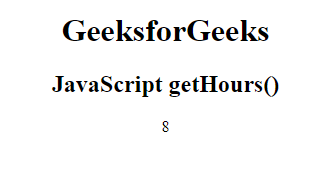getHours() Method

The getDate() Method: The getDate() method in JavaScript is used to return the day of a date as a number (1-31).

Example: This example describes the getDate() method for retrieving the current date of the month from the date object.

## HTML

 ```<``html``>` `<``head``>``    ``<``title``>JavaScript getDate() Method``` `<``body` `style``=``"text-align:center;"``>``    ``<``h1``>GeeksforGeeks``    ``<``h2``>JavaScript getDate()``    ``<``p` `id``=``"GFG"``>` `    ` `    ````    ``<``script``>``    ``var d = new Date();``    ``document.getElementById("GFG").innerHTML = d.getDate();``    ````` ``

Output: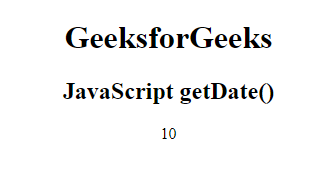getDate() Method

The getMonth() Method: The getMonth() method in JavaScript is used to return the month of a date as a number (0-11).

Example: This example describes the getMonth() method for retrieving the month from the date object.

## HTML

 ```<``html``>` `<``head``>``    ``<``title``>JavaScript getMonth() Method``` `<``body` `style``=``"text-align:center;"``>``    ``<``h1``>GeeksforGeeks``    ``<``h2``>JavaScript getMonth()``    ``<``p` `id``=``"GFG"``>` `    ` `    ````    ``<``script``>``    ``var d = new Date();``    ``document.getElementById("GFG").innerHTML = d.getMonth()+1;``    ````` ``

In Javascript, the month number starts from 0 which denotes 1st month ie., January & ends with the month number 11 which denotes the last month ie., December. So, we need to add 1 to get the current month.

Output: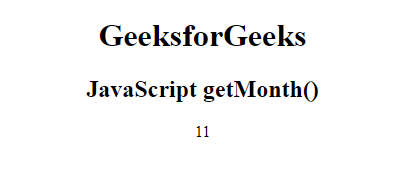getMonth() Method

The getFullYear() Method: The getFullYear() method in JavaScript is used to return the year (in 4-digit) for the specified date according to local time.

Example: This example describes the getFullYear() method for retrieving the year from the date object.

## HTML

 ```<``html``>` `<``head``>``    ``<``title``>JavaScript getFullYear() Method``` `<``body` `style``=``"text-align:center;"``>``    ``<``h1``>GeeksforGeeks``    ``<``h2``>JavaScript getFullYear()``    ``<``p` `id``=``"GFG"``>` `    ` `    ````    ``<``script``>``    ``var d = new Date();``    ``document.getElementById("GFG").innerHTML = d.getFullYear();``    ````` ``

Output: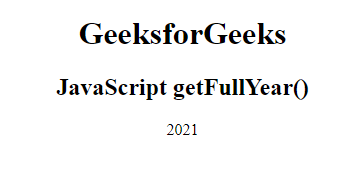getFullYear() Method

The getTime() Method: The getTime() method in JavaScript is used to return the number of milliseconds(0–999).

Example: This example describes the getTime() method for retrieving the number of milliseconds from the date object.

## HTML

 ```<``html``>` `<``head``>``    ``<``title``>JavaScript getTime() Method``` `<``body` `style``=``"text-align:center;"``>``    ``<``h1``>GeeksforGeeks``    ``<``h2``>JavaScript getTime()``    ``<``p` `id``=``"GFG"``>` `    ` `    ````    ``<``script``>``    ``var d = new Date();``    ``document.getElementById("GFG").innerHTML = d.getTime();``    ````` ``

Output: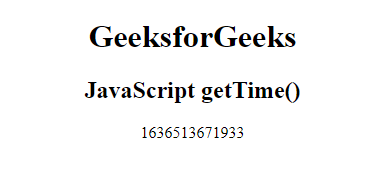getTime() Method

The getSeconds() Method: The getSeconds() method in JavaScript returns the seconds of a date object (0-59).

Example: This example describes the getSeconds() method for retrieving the seconds from the date object.

## HTML

 ```<``html``>` `<``head``>``    ``<``title``>JavaScript getSeconds() Method``` `<``body` `style``=``"text-align:center;"``>``    ``<``h1``>GeeksforGeeks``    ``<``h2``>JavaScript getSeconds()``    ``<``p` `id``=``"GFG"``>` `    ` `    ````    ``<``script``>``    ``var d = new Date();``    ``document.getElementById("GFG").innerHTML = d.getSeconds();``    ````` ``

Output: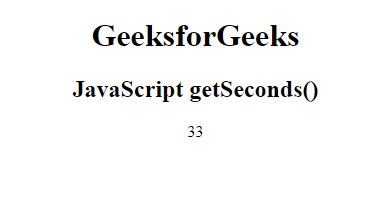getSeconds() Method

Supported Browsers: